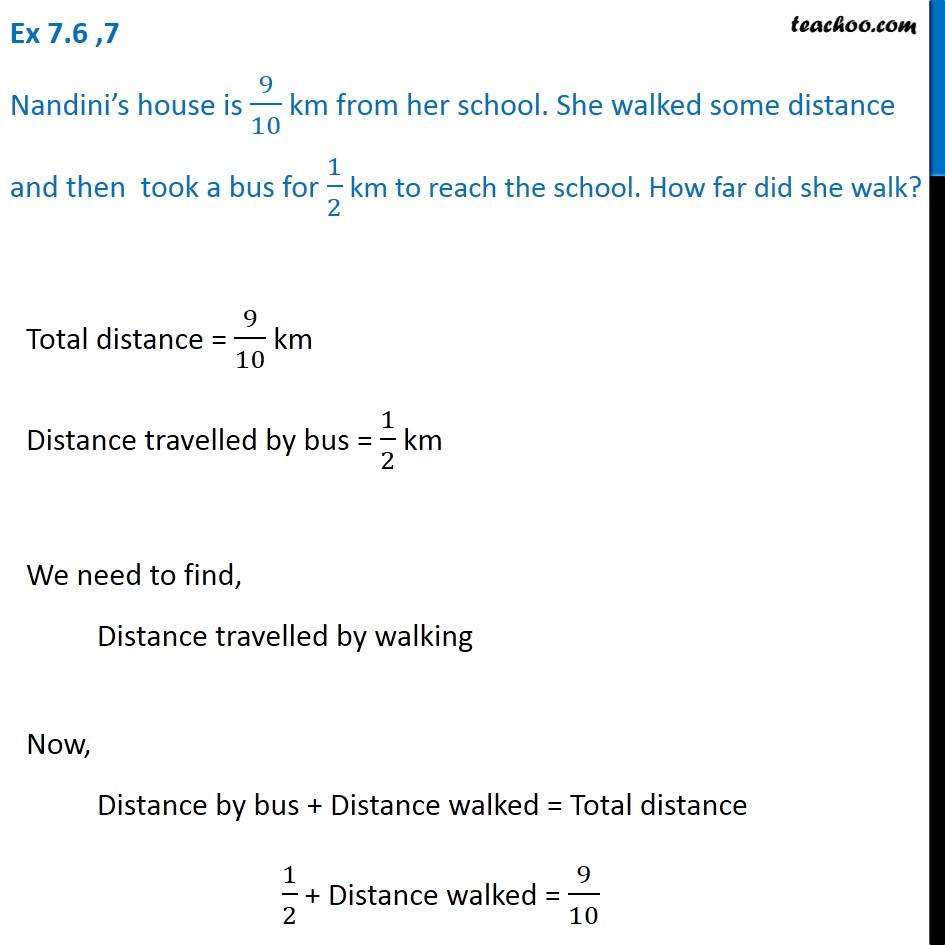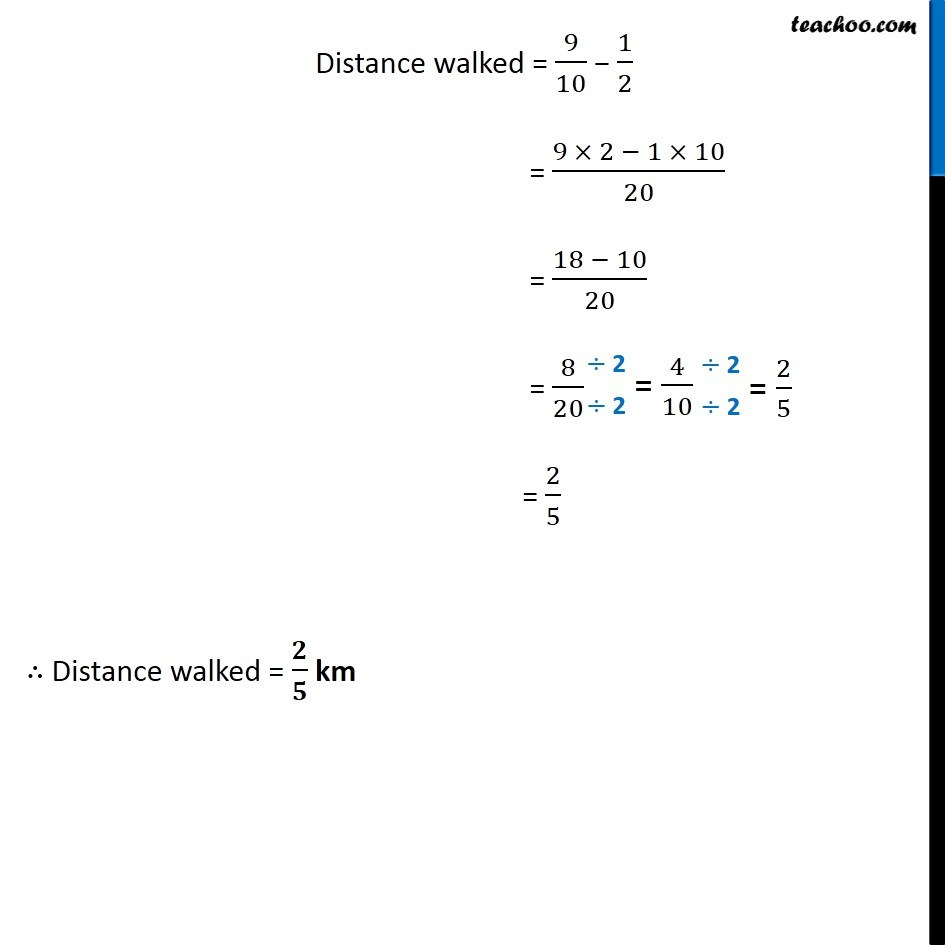Ex 7.6

Chapter 7 Class 6 Fractions
Serial order wiseLearn in your speed, with individual attention - Teachoo Maths 1-on-1 Class

### Transcript

Ex 7.6 ,7 Nandini’s house is 9/10 km from her school. She walked some distance and then took a bus for 1/2 km to reach the school. How far did she walk? Total distance = 9/10 km Distance travelled by bus = 1/2 km We need to find, Distance travelled by walking Now, Distance by bus + Distance walked = Total distance 1/2 + Distance walked = 9/10 Distance walked = 9/10 − 1/2 = (9 × 2 − 1 × 10)/20 = (18 − 10)/20 = 8/20 = 2/5 ∴ Distance walked = 𝟐/𝟓 km